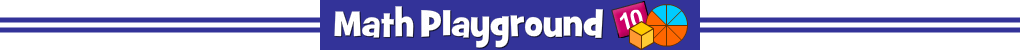Directions
Fraction Forest is a game of exploration. Part of the fun is figuring out what to do.

On Level 1, you have to place the correct number of fraction pieces on the table. If you know equivalent fractions, the ruler will help you find the exact number to place. Click to highlight fractions then click the table.

On many of the levels, the forest animal is thinking about the fraction it needs. Your job is to make the right size pieces and give them to the animal. Sometimes the animal will want one just one block. You may need to use the glue tool to combine smaller fraction pieces. TIP: To finish some levels, select the final fraction piece. Then select the animal or object that is asking for the fraction.

Want to practice more problems like this? Try Equivalent Fraction Playground.
Need a simpler challenge? Try Belle and Cody Part 1.
Belle and Cody Part 2 - Learning Connections
Essential Skills
Mental Math - solve fraction puzzles visually

Common Core Connections
Fraction 1/b is formed by 1 part when a whole is partitioned into b equal parts.
Fraction a/b is formed by a parts of size 1/b.
Compare fractions by reasoning about their size.
Explain why two fractions are equivalent by using a visual fraction model.
More Math Games to Play